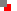﻿ Element Bezier

Element <B>

The element "B" is used to define any point of a Bezier curve.

To draw a Bezier curve, first define a starting point. The next two points are 'control points'; these points are not positioned on the line but are used to guide the curve to the next defined point, which is positioned on the line. Continue to define groups of three points (i.e. 2 off-line control points + 1 on-line position point) until the line is drawn.

If the starting-point is omitted then the group origin is used.
If the start and end-points are both omitted then the group origin is used for both.Drawing elements sample script